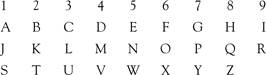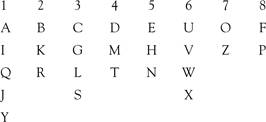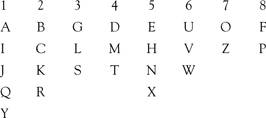# numerology

(redirected from Numberology)
Also found in: Dictionary, Thesaurus, Medical.

## numerology

the study of numbers, such as the figures in a birth date, and of their supposed influence on human affairs
Collins Discovery Encyclopedia, 1st edition © HarperCollins Publishers 2005

## Numerology

(religion, spiritualism, and occult)

Numerology is divination through the occult significance of numbers. Pythagoras said, “The world is built upon the power of numbers.” He was the one who reduced all to the power of the nine primaries. The numerals 1 through 9 are therefore the basis of all numbers and calculations in numerology. This is done by adding anything above 9. For example, 26 = 2+6 = 8. No matter the size of the number, it can be reduced in the same way. For example: 7,548,327 would become 7+5+4+8+3+2+7 = 36 = 3+6 = 9.

Working in Numerology, the same reduction can take place with words and letters, based on the following:The word CASTLE, for example, would have the values C=3, A=1, S= 1, T=2, L=3, E=5; 3+1 +1+2+3+5 = 15 = 1+5 = 6. So the numerological value for the word “Castle” is 6.

In Numerology, dates and names are reduced to their primary numbers and then interpreted according to the values attributed to those numbers. Working with this, you can determine everything from where would be best to live, what job would be best for you, what sort of person to marry, and so on. To do this, it is first necessary to find your Birth Number and your Name Number.

For someone born on August 27, 1986, their birth number would be that date reduced to a single digit: 8+2+7 + 1+9+8+6 = 41 = 4+1 = 5. That person’s Birth Number is 5. This number represents the influences at the time of birth and is the number to consider for all important events—such as signing contracts, or getting married. Since it is the number of your birth, it cannot be altered; unlike a Name Number which can be altered.

The Name Number is taken from the above chart. For example, Elvis Presley had a name number that was 5+3+4+9+1+7+9+5+1+3+5+7 = 59 = 14 = 5. Notice the 5s that occur. 5 people are physically and mentally active, make friends easily, and get along well with almost any other number. They are usually leaders of one sort or another. Another even more striking example of 5 was John F. Kennedy: 1685 6 2555547 = 59 = 14 = 5. Here there is a tremendous preponderance of 5s, ending with the Name Number itself being 5. Any recurring numeral, such as this, is considered to affect the personality.

The name you reduce in this way should be the name most generally used. Hence, “John F Kennedy” rather than just “John Kennedy” or “John Fitzgerald Kennedy.” Based on this, Elvis’s stronger Name Number would be simply ELVIS = 5+3+4+9+1 = 24 = 6; the 5 would be a secondary Name Number, contributing some qualities but not the major ones. Marriage compatibility can be seen by comparing birth numbers and considering name numbers.

There are certain values that are traditionally attached to the nine primary numbers. For example, 1 (Letters A, J, S) is associated with the Sun, and with the element of Fire. It is also associated with a leader and driving force who automatically assumes control. Each of the numbers has such associations.

There are also other systems of Numerology. All seem workable, but it is not wise to mix different systems. The above system is the most commonly used, and is referred to as the “modern” system or the “Pythagorean” system. The Hebrew system does not use the number 9, and does not follow the normal arrangement of letters. The Hebrew equivalents of English letters have been used, except where there is no exact parallel:Another system is the one devised and used by Samuel Liddell MacGregor Mathers, of the nineteenth century Order of the Golden Dawn:Sources:

Buckland, Raymond: The Fortune–Telling Book: The Encyclopedia of Divination and Soothsaying. Detroit: sible Ink Press, 2004
Cavendish, Marshall: The Book of Fate & Fortune. London: Cavendish House, 1981
Cheasley, Clifford W.: Numerology. Boston: Triangle, 1916
Cheiro (Louis Hamon): Cheiro’s Book of Numbers. New York: Arc, 1964
Leek, Sybil: Numerology: The Magic of Numbers. New York: Collier, 1969

Lopez, Vincent: Numerology. New York: Citadel, 1961